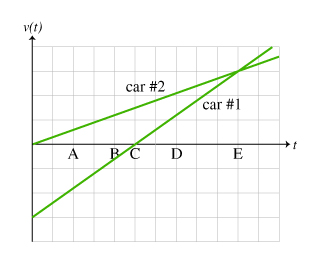# Problem: Two cars travel on the parallel lanes of a two-lane road. The cars are at the same location at time t=0s, and move in such a way as to produce the velocity (relative to the ground) vs. time graph shown in the figure. (Figure 1)On the graph, one vertical block is equivalent to one velocity unit.Rank car #1's speed relative to the ground at the lettered times (A through E).Rank from largest to smallest. To rank items as equivalent, overlap them.

###### FREE Expert Solution

The magnitude of the velocity (the speed) is given by the vertical distance from the time-axis.

vA = 1.75

vB = 0.5

vC = 0###### Problem Details

Two cars travel on the parallel lanes of a two-lane road. The cars are at the same location at time t=0s, and move in such a way as to produce the velocity (relative to the ground) vs. time graph shown in the figure. (Figure 1)On the graph, one vertical block is equivalent to one velocity unit.Rank car #1's speed relative to the ground at the lettered times (A through E).

Rank from largest to smallest. To rank items as equivalent, overlap them.